# CONTENTS Loop Statements Parts of a loop Types

• Slides: 39CONTENTS Loop Statements Parts of a loop Types of Loops 1. 2. 3. § § § 4. 5. 6. While Loop For Loop Do-while Loop Nested Loops Jump Statements Exercises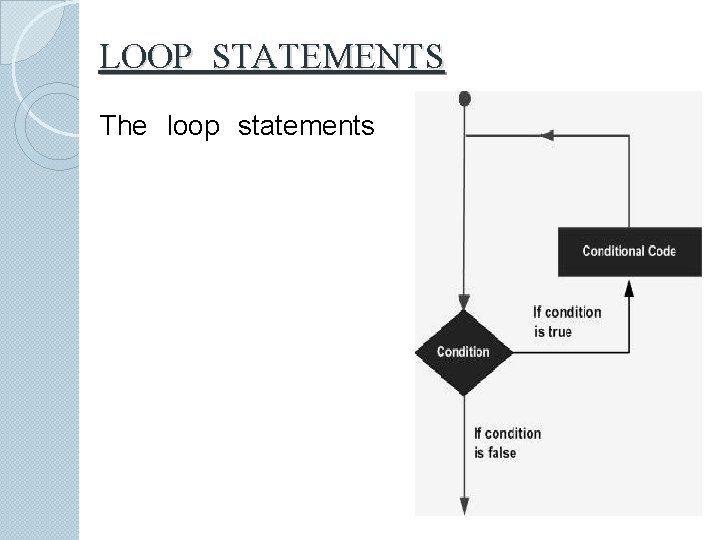LOOP STATEMENTS The loop statements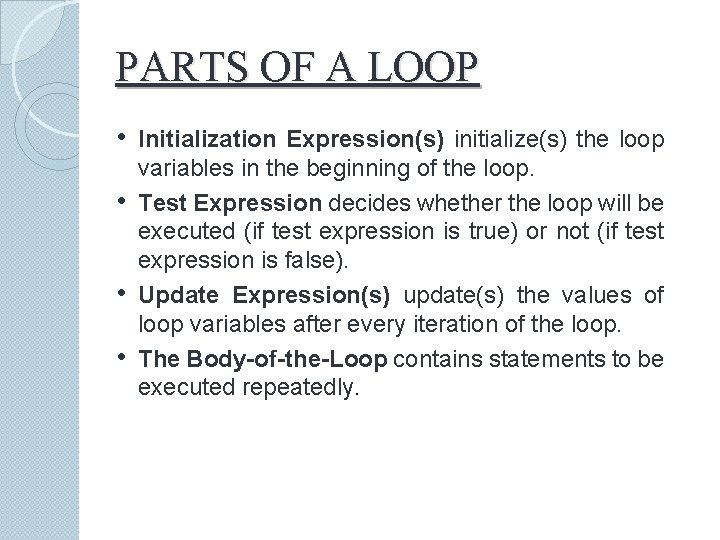PARTS OF A LOOP • Initialization Expression(s) initialize(s) the loop • • • variables in the beginning of the loop. Test Expression decides whether the loop will be executed (if test expression is true) or not (if test expression is false). Update Expression(s) update(s) the values of loop variables after every iteration of the loop. The Body-of-the-Loop contains statements to be executed repeatedly.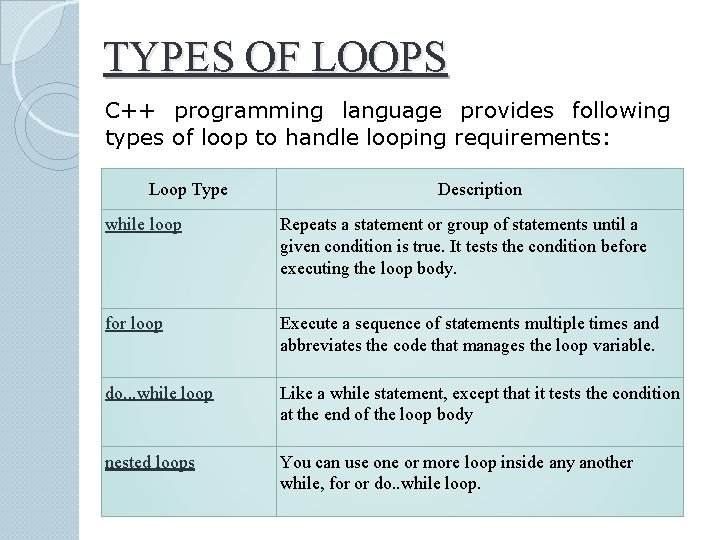TYPES OF LOOPS C++ programming language provides following types of loop to handle looping requirements: Loop Type Description while loop Repeats a statement or group of statements until a given condition is true. It tests the condition before executing the loop body. for loop Execute a sequence of statements multiple times and abbreviates the code that manages the loop variable. do. . . while loop Like a while statement, except that it tests the condition at the end of the loop body nested loops You can use one or more loop inside any another while, for or do. . while loop.WHILE LOOP • The syntax of while statement : while (loop repetition condition) condition statement • Loop repetition condition is the condition which controls the loop. • The statement is repeated as long as the loop repetition condition is true • A loop is called an infinite loop if the loop repetition condition is always true.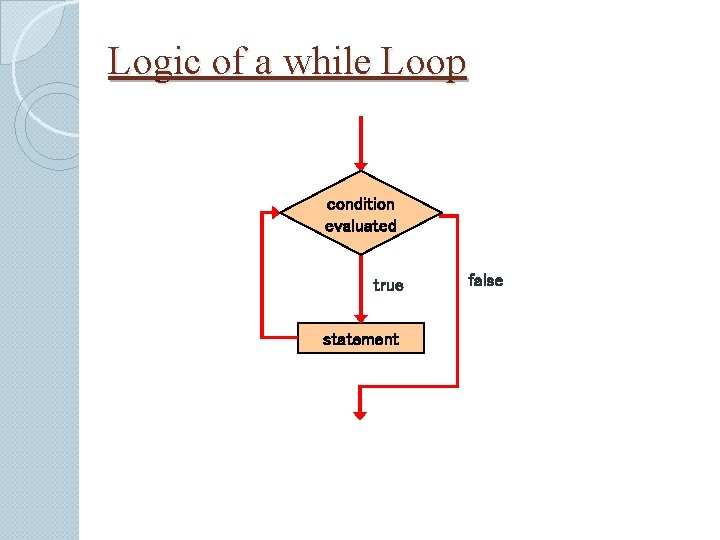Logic of a while Loop condition evaluated true statement false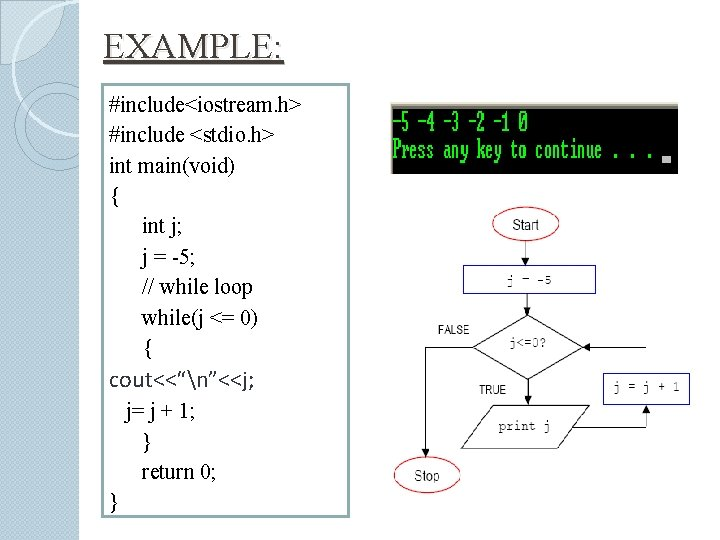EXAMPLE: #include<iostream. h> #include <stdio. h> int main(void) { int j; j = -5; // while loop while(j <= 0) { cout<<“n”<<j; j= j + 1; } return 0; }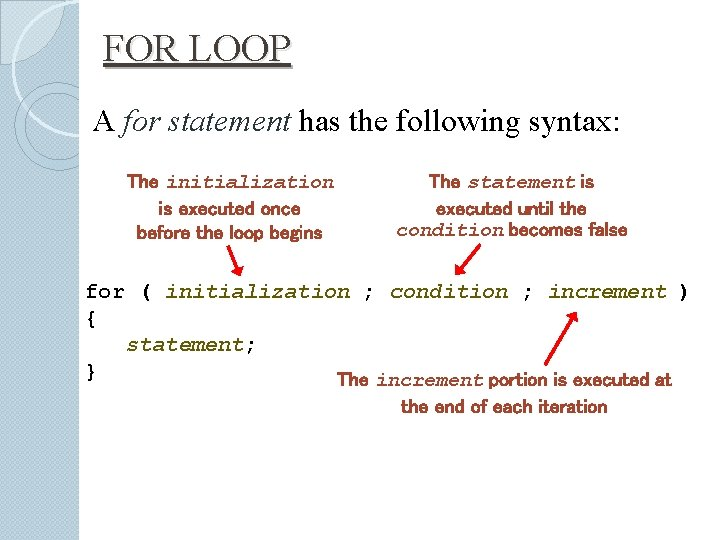FOR LOOP A for statement has the following syntax: The initialization is executed once before the loop begins The statement is executed until the condition becomes false for ( initialization ; condition ; increment ) { statement; } The increment portion is executed at the end of each iterationLogic of a for loop initialization condition evaluated true statement increment false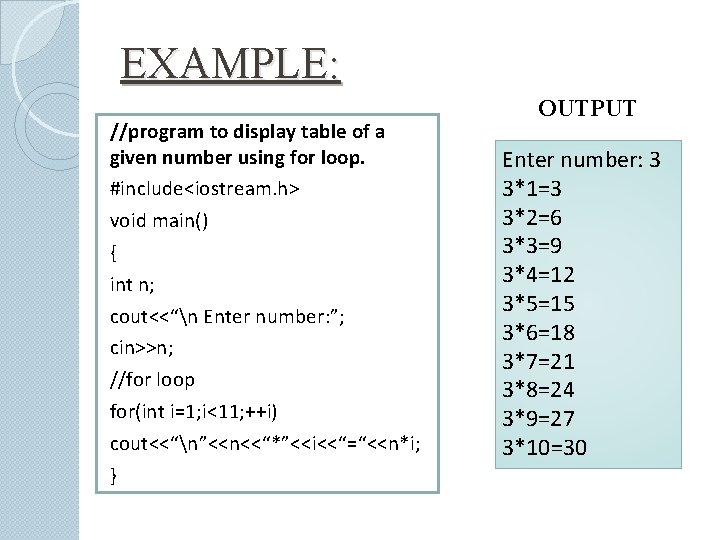EXAMPLE: //program to display table of a given number using for loop. #include<iostream. h> void main() { int n; cout<<“n Enter number: ”; cin>>n; //for loop for(int i=1; i<11; ++i) cout<<“n”<<n<<“*”<<i<<“=“<<n*i; } OUTPUT Enter number: 3 3*1=3 3*2=6 3*3=9 3*4=12 3*5=15 3*6=18 3*7=21 3*8=24 3*9=27 3*10=30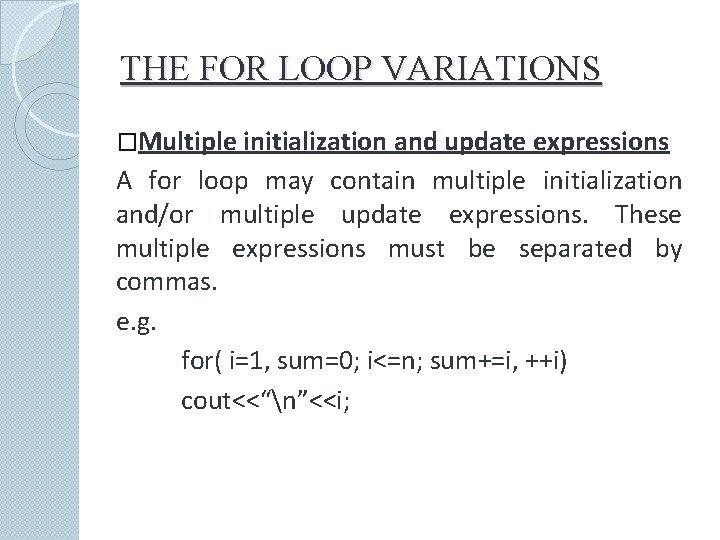THE FOR LOOP VARIATIONS �Multiple initialization and update expressions A for loop may contain multiple initialization and/or multiple update expressions. These multiple expressions must be separated by commas. e. g. for( i=1, sum=0; i<=n; sum+=i, ++i) cout<<“n”<<i;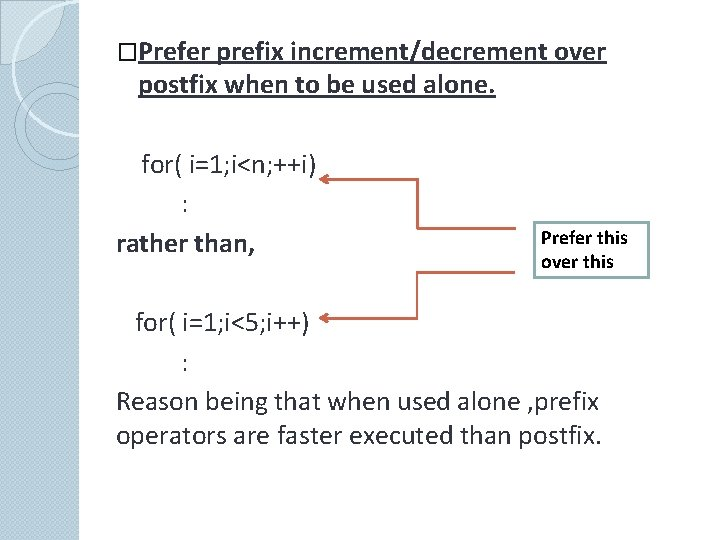�Prefer prefix increment/decrement over postfix when to be used alone. for( i=1; i<n; ++i) : rather than, Prefer this over this for( i=1; i<5; i++) : Reason being that when used alone , prefix operators are faster executed than postfix.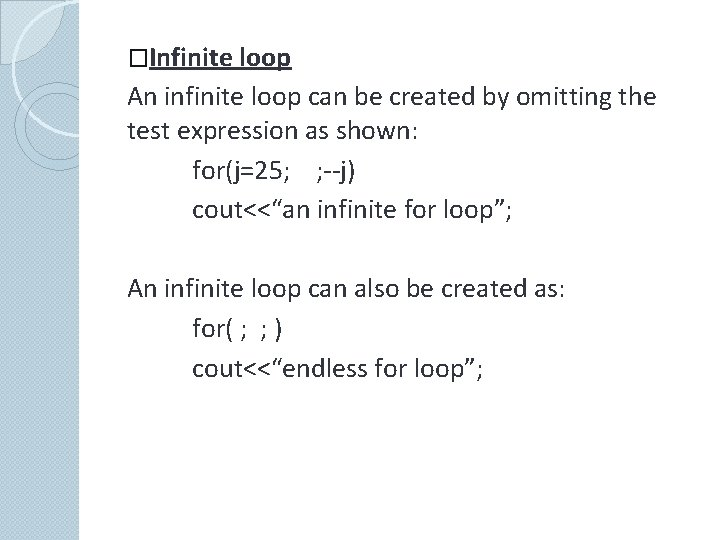�Infinite loop An infinite loop can be created by omitting the test expression as shown: for(j=25; ; --j) cout<<“an infinite for loop”; An infinite loop can also be created as: for( ; ; ) cout<<“endless for loop”;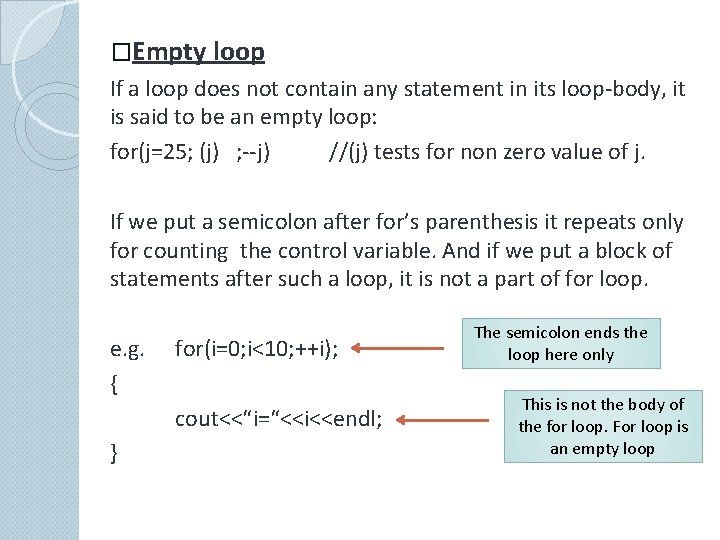�Empty loop If a loop does not contain any statement in its loop-body, it is said to be an empty loop: for(j=25; (j) ; --j) //(j) tests for non zero value of j. If we put a semicolon after for’s parenthesis it repeats only for counting the control variable. And if we put a block of statements after such a loop, it is not a part of for loop. e. g. { for(i=0; i<10; ++i); cout<<“i=“<<i<<endl; } The semicolon ends the loop here only This is not the body of the for loop. For loop is an empty loop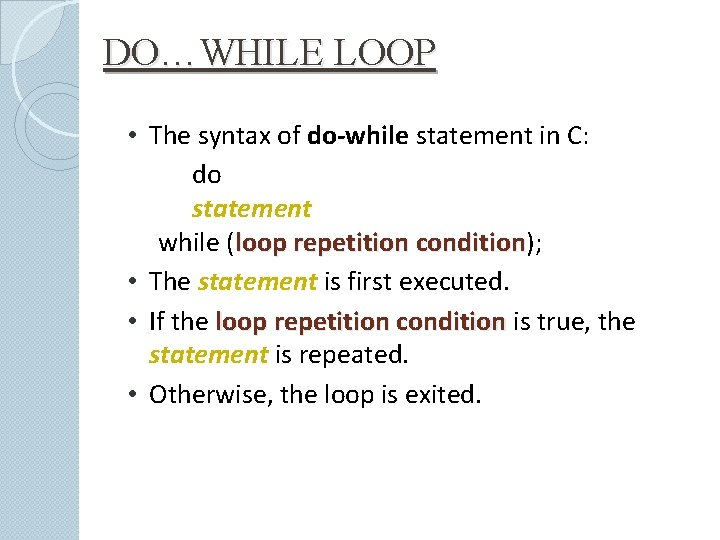DO…WHILE LOOP • The syntax of do-while statement in C: do statement while (loop repetition condition); condition • The statement is first executed. • If the loop repetition condition is true, the statement is repeated. • Otherwise, the loop is exited.Logic of a do…while loop statement true condition evaluated false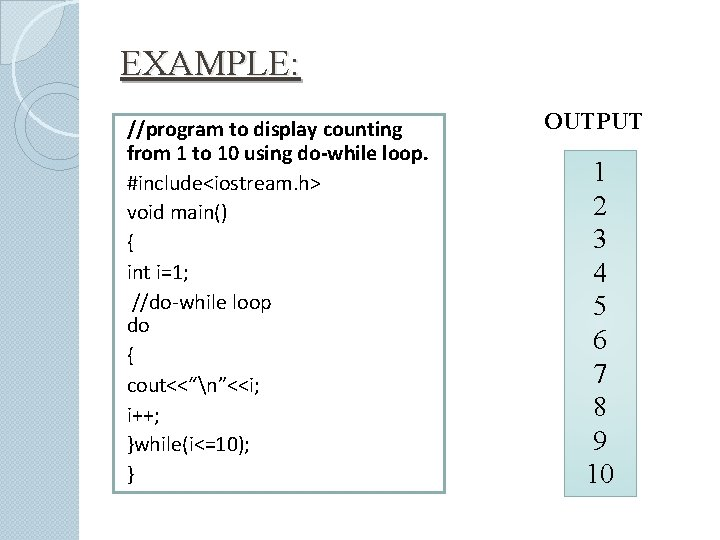EXAMPLE: //program to display counting from 1 to 10 using do-while loop. #include<iostream. h> void main() { int i=1; //do-while loop do { cout<<“n”<<i; i++; }while(i<=10); } OUTPUT 1 2 3 4 5 6 7 8 9 10NESTED LOOPS Nested loops consist of an outer loop with one or more inner loops e. g. , Outer loop for (i=1; i<=100; i++){ for(j=1; j<=50; j++){ … Inner loop } } • The above loop will run for 100*50 iterations. •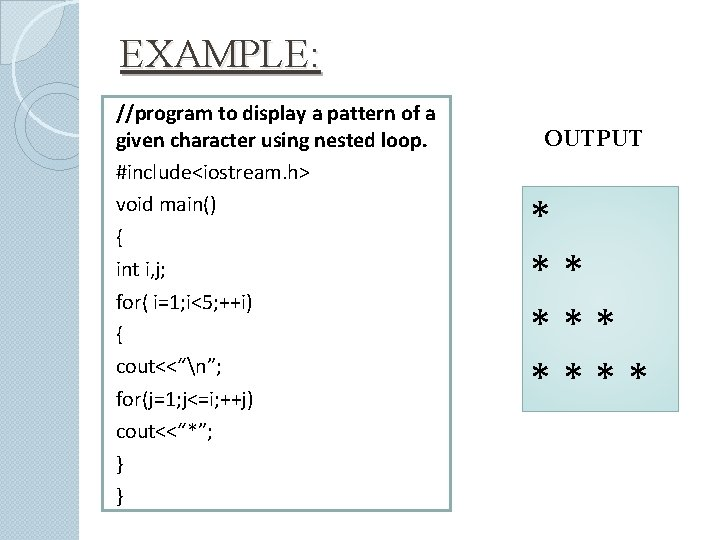EXAMPLE: //program to display a pattern of a given character using nested loop. #include<iostream. h> void main() { int i, j; for( i=1; i<5; ++i) { cout<<“n”; for(j=1; j<=i; ++j) cout<<“*”; } } OUTPUT * ** ****JUMP STATEMENTS 1. The goto statement • A goto statement can transfer the program control anywhere in the program. • The target destination is marked by a label. • The target label and goto must appear in the same statement. • The syntax of goto statement is: goto label; … label: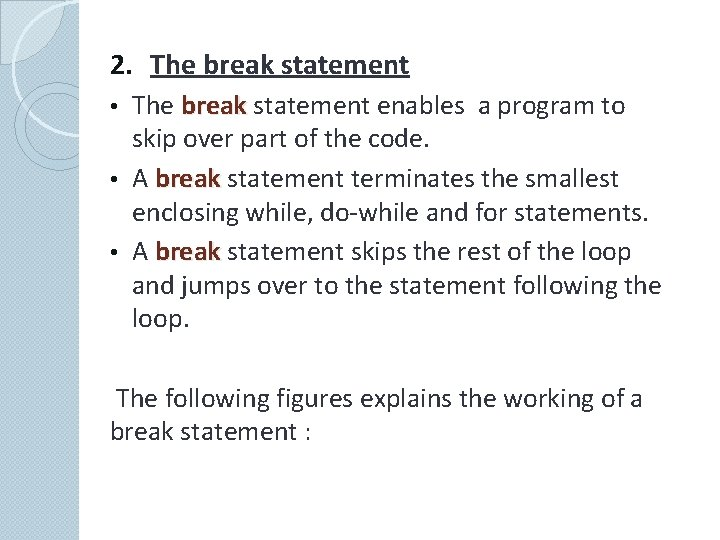2. The break statement enables a program to skip over part of the code. • A break statement terminates the smallest enclosing while, do-while and for statements. • A break statement skips the rest of the loop and jumps over to the statement following the loop. • The following figures explains the working of a break statement :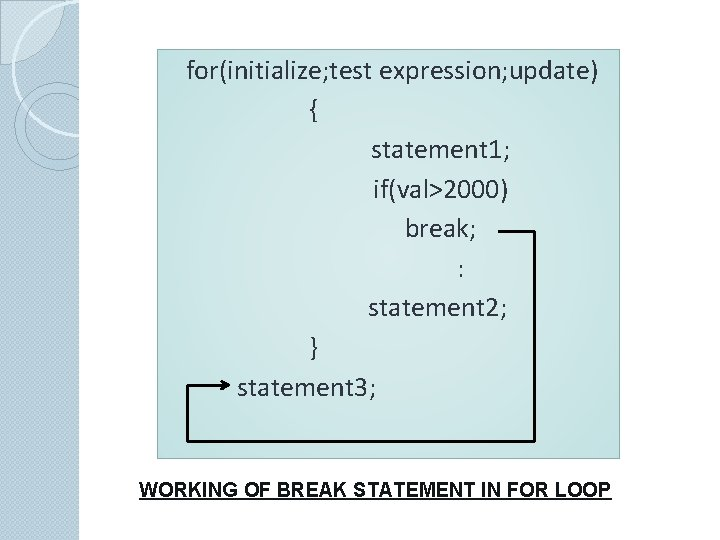for(initialize; test expression; update) { statement 1; if(val>2000) break; : statement 2; } statement 3; WORKING OF BREAK STATEMENT IN FOR LOOPwhile(test expression) { statement 1; if(val>2000) break; : statement 2; } statement 3; WORKING OF BREAK STATEMENT IN WHILE LOOP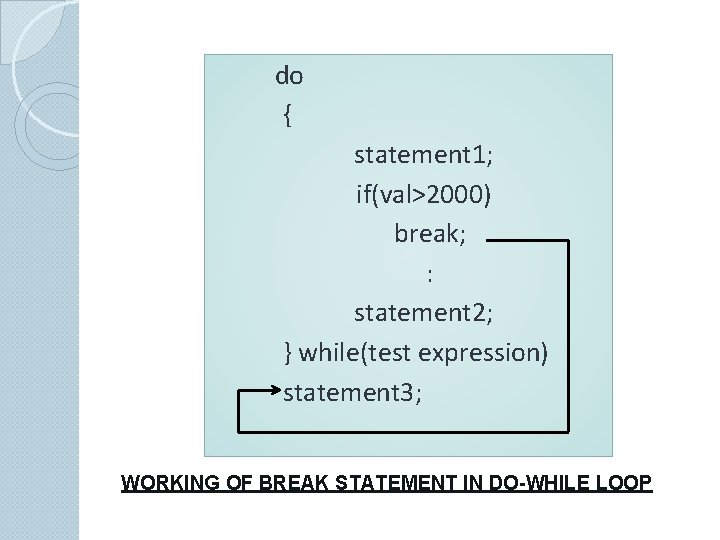do { statement 1; if(val>2000) break; : statement 2; } while(test expression) statement 3; WORKING OF BREAK STATEMENT IN DO-WHILE LOOP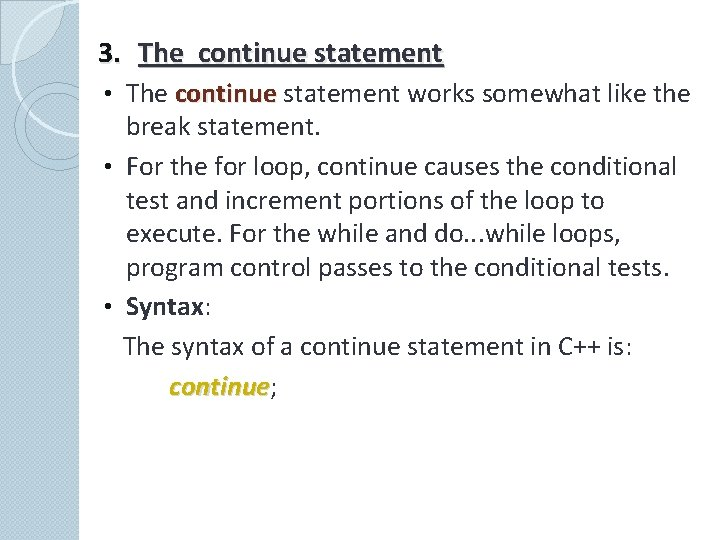3. The continue statement • The continue statement works somewhat like the break statement. • For the for loop, continue causes the conditional test and increment portions of the loop to execute. For the while and do. . . while loops, program control passes to the conditional tests. • Syntax: The syntax of a continue statement in C++ is: continue; continue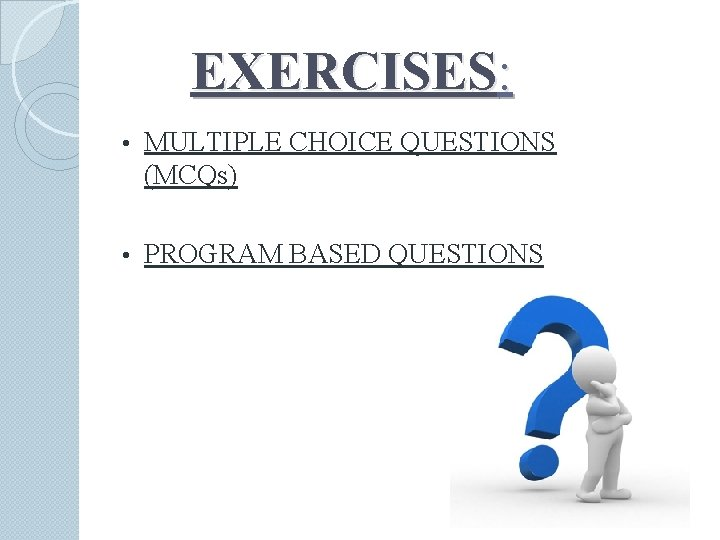EXERCISES: • MULTIPLE CHOICE QUESTIONS (MCQs) • PROGRAM BASED QUESTIONSMCQs. . .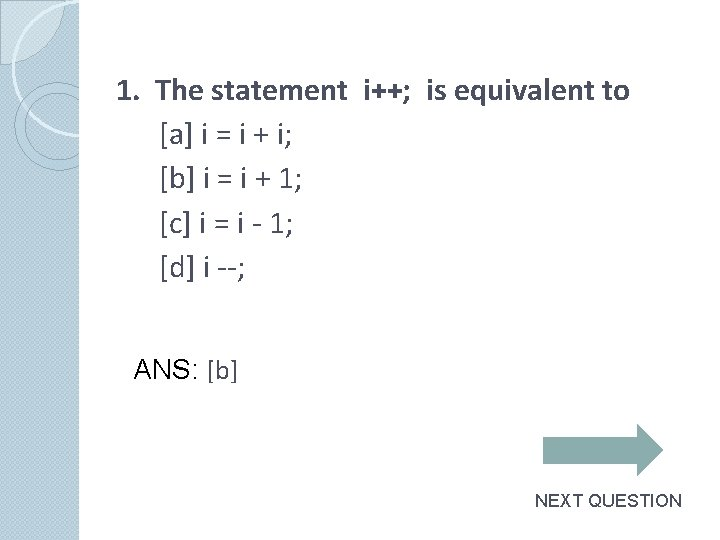1. The statement i++; is equivalent to [a] i = i + i; [b] i = i + 1; [c] i = i - 1; [d] i --; ANS: [b] NEXT QUESTION2. What's wrong? for (int k = 2, k <=12, k++) [a] the increment should always be ++k [b] the variable must always be the letter i when using a for loop [c] there should be a semicolon at the end of the statement [d] the commas should be semicolons ANS: [d] NEXT QUESTION3. Which looping process checks the test condition at the end of the loop? [a] for [b] while [c] do-while [d] no looping process checks the test condition at the end ANS: [c] NEXT QUESTION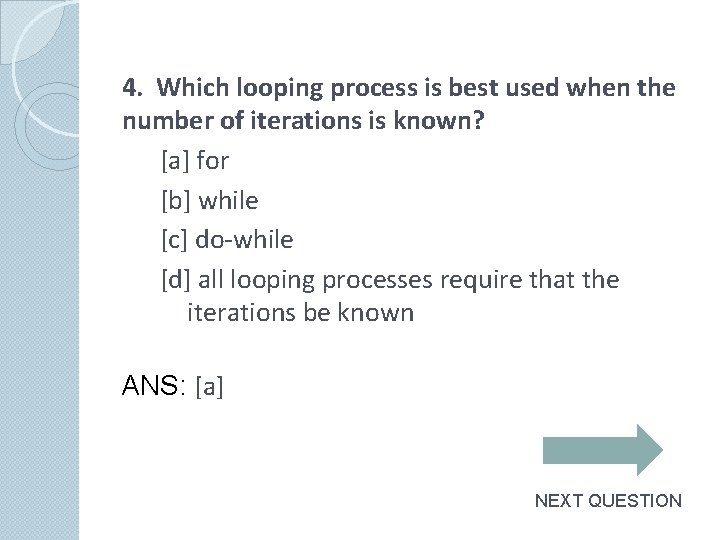4. Which looping process is best used when the number of iterations is known? [a] for [b] while [c] do-while [d] all looping processes require that the iterations be known ANS: [a] NEXT QUESTION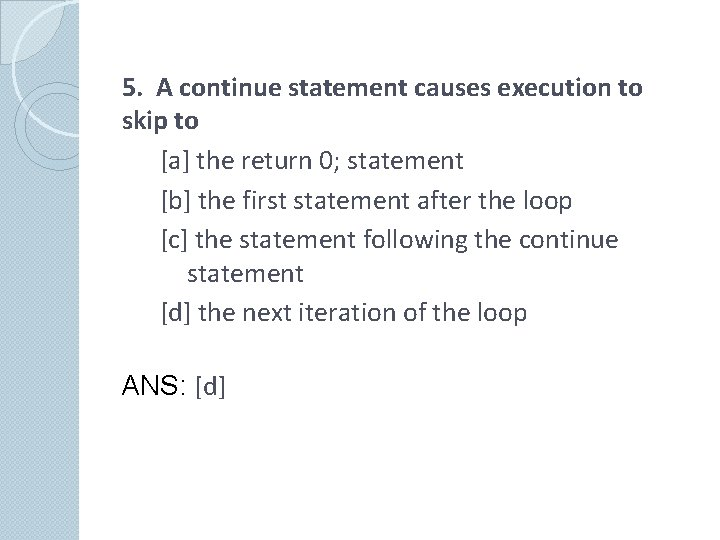5. A continue statement causes execution to skip to [a] the return 0; statement [b] the first statement after the loop [c] the statement following the continue statement [d] the next iteration of the loop ANS: [d]PROGRAM BASED QUESTIONS…1. Write a program to print first n natural numbers and their sum. 2. Write a program to calculate the factorial of an integer. 3. Write a program that prints 1 2 4 8 16 32 64 128. 4. Write a program to check whether the given number is palindrome or not. 5. Write a program to generate divisors of an integer. 6. Write a program to find whether a given number is odd or even. The program should continue as long as the user wants. 7. Write a program to print Fibonacci series i. e. , 0 1 1 2 3 5 8….8. Write a program to print largest even and largest odd number from a list of numbers entered. The list terminates as soon as one enters 0(zero). 9. Write a program to check whether the entered number is Armstrong or not. 10. Write a program to display Pythagorean triplets up to 100. 11. Write a program to calculate average of 10 numbers. 12. Write programs to produce the following designs: a) A A B C D E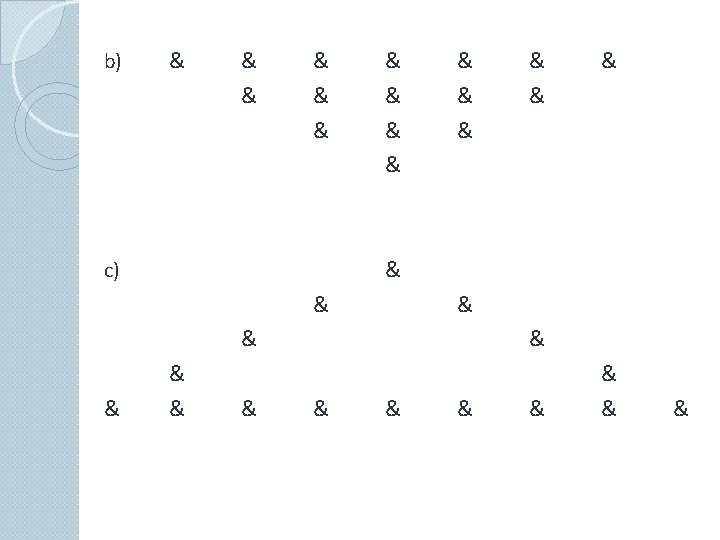THANK YOU